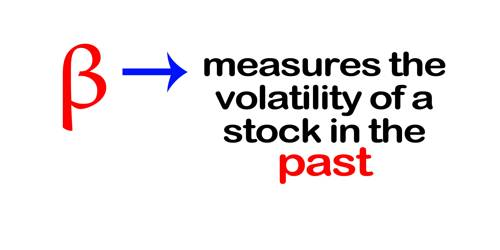# Concept of Stock of Beta

The concept of Stock of Beta

A stock of beta coefficient, ‘β’ is a measure of the stock’s market risk. Beta measures the volatility of returns on a security relative to returns on the market, which is the portfolio of all risky assets. It is a numeric value that measures the fluctuations of stock to changes in the overall stock market. Beta is used in the capital asset pricing model (CAPM), which calculates the expected return of an asset based on its beta and expected market returns. While beta is a measure of relative market risk, the actual market risk of stock ‘J’ is ‘β’ Stock J’s stand-alone risk is the beta coefficient is measured by the slope of the stock’s characteristic line, which is found by regressing historical returns on the stock versus the historical returns on the market. A high beta stock is more volatile than an average stock, while a low-beta stock is less volatile than average. The beta coefficient assumes that stock returns are normally distributed from a statistical perspective. An average beta stock has β = 1.0, by definition. The beta of a portfolio is a weighted average of the betas of the individual securities in the portfolio.

For example, if a stock’s beta value is 1.3, it means, theoretically this stock is 30% more volatile than the market. A company with a higher beta has greater risk and also greater expected returns. The basic thought is that the higher your beta is, the riskier the stock is.